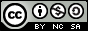- 目录 -
LeetCode 572 另一个树的子树(dfs,树哈希)

     3
/ \
4   5
/ \
1   2


   4
/ \
1   2


     3
/ \
4   5
/ \
1   2
/
0


   4
/ \
1   2


## 代码

  1 2 3 4 5 6 7 8 9 10 11 12 13 14 15 16 17 18 19 20 21 22 23 24 25 26 27 28 29 30 31 32 33 34 35 36 37 38 39  class Solution { public: // 判断当前的s树是否被t树包含 bool dfs(TreeNode *s, TreeNode *t) { if (!s || !t) return false; if (s->val != t->val) return false; bool f1 = true, f2 = true; if (s->left) f1 = dfs(s->left, t->left); if (s->right) f2 = dfs(s->right, t->right); if (f1 && f2) return true; return false; } bool isSubtree(TreeNode *s, TreeNode *t) { if (!s && !t) return true; queue q; q.push(s); while (!q.empty()) { TreeNode *node = q.front(); q.pop(); if (dfs(node, t) && dfs(t, node)) return true; if (node->left) q.push(node->left); if (node->right) q.push(node->right); } return false; } }; 

  1 2 3 4 5 6 7 8 9 10 11 12 13 14 15 16 17 18 19 20 21  class Solution { public: // 判断s树是否等于t树 bool dfs(TreeNode *s, TreeNode *t) { if (!s && !t) return true; if ((!s && t) || (s && !t)) return false; return (s->val == t->val) && dfs(s->left, t->left) && dfs(s->right, t->right); } bool isSubtree(TreeNode *s, TreeNode *t) { if (!s) return false; if (dfs(s, t)) return true; return isSubtree(s->left, t) || isSubtree(s->right, t); } }; 

  1 2 3 4 5 6 7 8 9 10 11 12 13 14 15 16 17 18 19 20 21 22 23 24 25 26 27 28 29 30 31 32 33 34 35 36 37 38 39  class Solution { public: // 存储以当前点为根的前序遍历序列 unordered_map mp; // 记录序列的数量 unordered_map scnt; void dfs(TreeNode *s) { mp[s] += to_string(s->val); if (!s->left && !s->right) { scnt[mp[s]]++; return; } if (s->left) { dfs(s->left); mp[s] += mp[s->left]; } if (s->right) { dfs(s->right); mp[s] += mp[s->right]; } scnt[mp[s]]++; } bool isSubtree(TreeNode *s, TreeNode *t) { if (!s && !t) return true; dfs(s); dfs(t); if (scnt[mp[t]] >= 2) return true; return false; } };